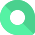# Gross Margin

## What is Gross Margin?

Gross Margin is a profitability ratio that measures Gross Profit as a percentage of total revenue. Typically, it is calculated as Gross Profit divided by revenue.

Alternate names: Gross Profit Margin, Markup

### How to calculate Gross Margin

ƒ Sum(Gross Profit) / Sum(Revenue)

### Example

If a florist has a revenue of \$15,000 and Cost of Goods Sold is \$6,000, their Gross Margin will be: (\$15,000 - \$6,000) / \$15,000 = 60%